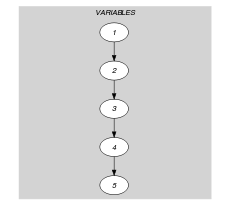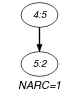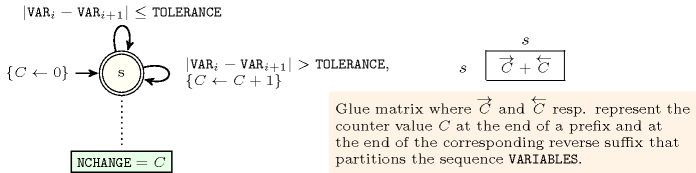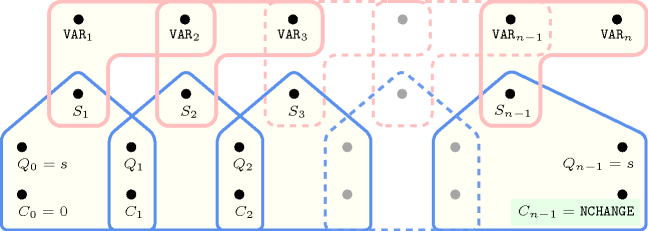## 5.355. smooth

Origin

Derived from $\mathrm{𝚌𝚑𝚊𝚗𝚐𝚎}$.

Constraint

$\mathrm{𝚜𝚖𝚘𝚘𝚝𝚑}\left(\mathrm{𝙽𝙲𝙷𝙰𝙽𝙶𝙴},\mathrm{𝚃𝙾𝙻𝙴𝚁𝙰𝙽𝙲𝙴},\mathrm{𝚅𝙰𝚁𝙸𝙰𝙱𝙻𝙴𝚂}\right)$

Arguments
 $\mathrm{𝙽𝙲𝙷𝙰𝙽𝙶𝙴}$ $\mathrm{𝚍𝚟𝚊𝚛}$ $\mathrm{𝚃𝙾𝙻𝙴𝚁𝙰𝙽𝙲𝙴}$ $\mathrm{𝚒𝚗𝚝}$ $\mathrm{𝚅𝙰𝚁𝙸𝙰𝙱𝙻𝙴𝚂}$ $\mathrm{𝚌𝚘𝚕𝚕𝚎𝚌𝚝𝚒𝚘𝚗}\left(\mathrm{𝚟𝚊𝚛}-\mathrm{𝚍𝚟𝚊𝚛}\right)$
Restrictions
 $\mathrm{𝙽𝙲𝙷𝙰𝙽𝙶𝙴}\ge 0$ $\mathrm{𝙽𝙲𝙷𝙰𝙽𝙶𝙴}<|\mathrm{𝚅𝙰𝚁𝙸𝙰𝙱𝙻𝙴𝚂}|$ $\mathrm{𝚃𝙾𝙻𝙴𝚁𝙰𝙽𝙲𝙴}\ge 0$ $\mathrm{𝚛𝚎𝚚𝚞𝚒𝚛𝚎𝚍}$$\left(\mathrm{𝚅𝙰𝚁𝙸𝙰𝙱𝙻𝙴𝚂},\mathrm{𝚟𝚊𝚛}\right)$
Purpose

$\mathrm{𝙽𝙲𝙷𝙰𝙽𝙶𝙴}$ is the number of times that $|X-Y|>\mathrm{𝚃𝙾𝙻𝙴𝚁𝙰𝙽𝙲𝙴}$ holds; $X$ and $Y$ correspond to consecutive variables of the collection $\mathrm{𝚅𝙰𝚁𝙸𝙰𝙱𝙻𝙴𝚂}$.

Example
$\left(1,2,〈1,3,4,5,2〉\right)$

In the example we have one change between values 5 and 2 since the difference in absolute value is greater than the tolerance (i.e., $|5-2|>2$). Consequently the $\mathrm{𝙽𝙲𝙷𝙰𝙽𝙶𝙴}$ argument is fixed to 1 and the $\mathrm{𝚜𝚖𝚘𝚘𝚝𝚑}$ constraint holds.

Typical
 $\mathrm{𝚃𝙾𝙻𝙴𝚁𝙰𝙽𝙲𝙴}>0$ $|\mathrm{𝚅𝙰𝚁𝙸𝙰𝙱𝙻𝙴𝚂}|>3$ $\mathrm{𝚛𝚊𝚗𝚐𝚎}$$\left(\mathrm{𝚅𝙰𝚁𝙸𝙰𝙱𝙻𝙴𝚂}.\mathrm{𝚟𝚊𝚛}\right)>1$
Symmetries
• Items of $\mathrm{𝚅𝙰𝚁𝙸𝙰𝙱𝙻𝙴𝚂}$ can be reversed.

• One and the same constant can be added to the $\mathrm{𝚟𝚊𝚛}$ attribute of all items of $\mathrm{𝚅𝙰𝚁𝙸𝙰𝙱𝙻𝙴𝚂}$.

Arg. properties
• Functional dependency: $\mathrm{𝙽𝙲𝙷𝙰𝙽𝙶𝙴}$ determined by $\mathrm{𝚃𝙾𝙻𝙴𝚁𝙰𝙽𝙲𝙴}$ and $\mathrm{𝚅𝙰𝚁𝙸𝙰𝙱𝙻𝙴𝚂}$.

• Prefix-contractible wrt. $\mathrm{𝚅𝙰𝚁𝙸𝙰𝙱𝙻𝙴𝚂}$ when $\mathrm{𝙽𝙲𝙷𝙰𝙽𝙶𝙴}=0$.

• Suffix-contractible wrt. $\mathrm{𝚅𝙰𝚁𝙸𝙰𝙱𝙻𝙴𝚂}$ when $\mathrm{𝙽𝙲𝙷𝙰𝙽𝙶𝙴}=0$.

• Prefix-contractible wrt. $\mathrm{𝚅𝙰𝚁𝙸𝙰𝙱𝙻𝙴𝚂}$ when $\mathrm{𝙽𝙲𝙷𝙰𝙽𝙶𝙴}=|\mathrm{𝚅𝙰𝚁𝙸𝙰𝙱𝙻𝙴𝚂}|-1$.

• Suffix-contractible wrt. $\mathrm{𝚅𝙰𝚁𝙸𝙰𝙱𝙻𝙴𝚂}$ when $\mathrm{𝙽𝙲𝙷𝙰𝙽𝙶𝙴}=|\mathrm{𝚅𝙰𝚁𝙸𝙰𝙱𝙻𝙴𝚂}|-1$.

Usage

This constraint is useful for the following problems:

• Assume that $\mathrm{𝚅𝙰𝚁𝙸𝙰𝙱𝙻𝙴𝚂}$ corresponds to the number of people that work on consecutive weeks. One may not normally increase or decrease too drastically the number of people from one week to the next week. With the $\mathrm{𝚜𝚖𝚘𝚘𝚝𝚑}$ constraint you can state a limit on the number of drastic changes.

• Assume you have to produce a set of orders, each order having a specific attribute. You want to generate the orders in such a way that there is not a too big difference between the values of the attributes of two consecutive orders. If you cannot achieve this on two given specific orders, this would imply a set-up or a cost. Again, with the $\mathrm{𝚜𝚖𝚘𝚘𝚝𝚑}$ constraint, you can control this kind of drastic changes.

Algorithm

A first incomplete algorithm is described in [BeldiceanuCarlsson01]. The sketch of a filtering algorithm for the conjunction of the $\mathrm{𝚜𝚖𝚘𝚘𝚝𝚑}$ and the $\mathrm{𝚜𝚝𝚛𝚎𝚝𝚌𝚑}$ constraints based on dynamic programming achieving arc-consistency is mentioned by Lars Hellsten in [Hellsten04].

Reformulation

The $\mathrm{𝚜𝚖𝚘𝚘𝚝𝚑}$ constraint can be reformulated with the $\mathrm{𝚜𝚎𝚚}_\mathrm{𝚋𝚒𝚗}$ constraint [PetitBeldiceanuLorca11] that we now introduce. Given $𝙽$ a domain variable, $𝚇$ a sequence of domain variables, and $𝙲$ and $𝙱$ two binary constraints, $\mathrm{𝚜𝚎𝚚}_\mathrm{𝚋𝚒𝚗}$$\left(𝙽,𝚇,𝙲,𝙱\right)$ holds if (1) $𝙽$ is equal to the number of $𝙲$-stretches in the sequence $𝚇$, and (2) $𝙱$ holds on any pair of consecutive variables in $𝚇$. A $𝙲$-stretch is a generalisation of the notion of stretch introduced by G. Pesant [Pesant01], where the equality constraint is made explicit by replacing it by a binary constraint $𝙲$, i.e., a $𝙲$-stretch is a maximal length subsequence of $𝚇$ for which the binary constraint $𝙲$ is satisfied on consecutive variables. $\mathrm{𝚜𝚖𝚘𝚘𝚝𝚑}$$\left(\mathrm{𝙽𝙲𝙷𝙰𝙽𝙶𝙴},\mathrm{𝚅𝙰𝚁𝙸𝙰𝙱𝙻𝙴𝚂},\mathrm{𝚃𝙾𝙻𝙴𝚁𝙰𝙽𝙲𝙴}\right)$ can be reformulated as $𝙽=𝙽\mathtt{1}-1\wedge$$\mathrm{𝚜𝚎𝚚}_\mathrm{𝚋𝚒𝚗}$$\left(𝙽\mathtt{1},𝚇,|{x}_{i}-{x}_{i+1}|\le \mathrm{𝚃𝙾𝙻𝙴𝚁𝙰𝙽𝙲𝙴},\mathrm{true}\right)$, where $\mathrm{true}$ is the universal constraint.

common keyword: $\mathrm{𝚌𝚑𝚊𝚗𝚐𝚎}$ (number of changes in a sequence with respect to a binary constraint).

Keywords
Arc input(s)

$\mathrm{𝚅𝙰𝚁𝙸𝙰𝙱𝙻𝙴𝚂}$

Arc generator
$\mathrm{𝑃𝐴𝑇𝐻}$$↦\mathrm{𝚌𝚘𝚕𝚕𝚎𝚌𝚝𝚒𝚘𝚗}\left(\mathrm{𝚟𝚊𝚛𝚒𝚊𝚋𝚕𝚎𝚜}\mathtt{1},\mathrm{𝚟𝚊𝚛𝚒𝚊𝚋𝚕𝚎𝚜}\mathtt{2}\right)$

Arc arity
Arc constraint(s)
$\mathrm{𝚊𝚋𝚜}\left(\mathrm{𝚟𝚊𝚛𝚒𝚊𝚋𝚕𝚎𝚜}\mathtt{1}.\mathrm{𝚟𝚊𝚛}-\mathrm{𝚟𝚊𝚛𝚒𝚊𝚋𝚕𝚎𝚜}\mathtt{2}.\mathrm{𝚟𝚊𝚛}\right)>\mathrm{𝚃𝙾𝙻𝙴𝚁𝙰𝙽𝙲𝙴}$
Graph property(ies)
$\mathrm{𝐍𝐀𝐑𝐂}$$=\mathrm{𝙽𝙲𝙷𝙰𝙽𝙶𝙴}$

Graph model

Parts (A) and (B) of Figure 5.355.1 respectively show the initial and final graph associated with the Example slot. Since we use the $\mathrm{𝐍𝐀𝐑𝐂}$ graph property, the unique arc of the final graph is stressed in bold.

##### Figure 5.355.1. Initial and final graph of the $\mathrm{𝚜𝚖𝚘𝚘𝚝𝚑}$ constraint(a) (b)
Automaton

Figure 5.355.2 depicts a first automaton that only accepts all the solutions to the $\mathrm{𝚜𝚖𝚘𝚘𝚝𝚑}$ constraint. This automaton uses a counter in order to record the number of satisfied constraints of the form $\left(|{\mathrm{𝚅𝙰𝚁}}_{i}-{\mathrm{𝚅𝙰𝚁}}_{i+1}|\right)>\mathrm{𝚃𝙾𝙻𝙴𝚁𝙰𝙽𝙲𝙴}$ already encountered. To each pair of consecutive variables $\left({\mathrm{𝚅𝙰𝚁}}_{i},{\mathrm{𝚅𝙰𝚁}}_{i+1}\right)$ of the collection $\mathrm{𝚅𝙰𝚁𝙸𝙰𝙱𝙻𝙴𝚂}$ corresponds a 0-1 signature variable ${S}_{i}$. The following signature constraint links ${\mathrm{𝚅𝙰𝚁}}_{i}$, ${\mathrm{𝚅𝙰𝚁}}_{i+1}$ and ${S}_{i}$: $\left(|{\mathrm{𝚅𝙰𝚁}}_{i}-{\mathrm{𝚅𝙰𝚁}}_{i+1}|\right)>\mathrm{𝚃𝙾𝙻𝙴𝚁𝙰𝙽𝙲𝙴}⇔{S}_{i}=1$.

##### Figure 5.355.2. Automaton (with one counter) of the $\mathrm{𝚜𝚖𝚘𝚘𝚝𝚑}$ constraint and its glue matrix##### Figure 5.355.3. Hypergraph of the reformulation corresponding to the automaton (with one counter) of the $\mathrm{𝚜𝚖𝚘𝚘𝚝𝚑}$ constraintSince the reformulation associated with the previous automaton is not Berge-acyclic, we now describe a second counter free automaton that also only accepts all the solutions to the $\mathrm{𝚜𝚖𝚘𝚘𝚝𝚑}$ constraint. Without loss of generality, assume that the collection of variables $\mathrm{𝚅𝙰𝚁𝙸𝙰𝙱𝙻𝙴𝚂}$ contains at least two variables (i.e., $|\mathrm{𝚅𝙰𝚁𝙸𝙰𝙱𝙻𝙴𝚂}|\ge 2$). Let $n$, $\mathrm{𝑚𝑖𝑛}$, $\mathrm{𝑚𝑎𝑥}$, and $𝒟$ respectively denote the number of variables of the collection $\mathrm{𝚅𝙰𝚁𝙸𝙰𝙱𝙻𝙴𝚂}$, the smallest value that can be assigned to the variables of $\mathrm{𝚅𝙰𝚁𝙸𝙰𝙱𝙻𝙴𝚂}$, the largest value that can be assigned to the variables of $\mathrm{𝚅𝙰𝚁𝙸𝙰𝙱𝙻𝙴𝚂}$, and the union of the domains of the variables of $\mathrm{𝚅𝙰𝚁𝙸𝙰𝙱𝙻𝙴𝚂}$. Clearly, the maximum number of changes (i.e., the number of times the constraint $\left(|{\mathrm{𝚅𝙰𝚁}}_{i}-{\mathrm{𝚅𝙰𝚁}}_{i+1}|\right)>\mathrm{𝚃𝙾𝙻𝙴𝚁𝙰𝙽𝙲𝙴}$ $\left(1\le i holds) cannot exceed the quantity $m=min\left(n-1,\overline{\mathrm{𝙽𝙲𝙷𝙰𝙽𝙶𝙴}}\right)$. The $\left(m+1\right)·|𝒟|+2$ states of the automaton that only accepts all the solutions to the $\mathrm{𝚜𝚖𝚘𝚘𝚝𝚑}$ constraint are defined in the following way:

• We have an initial state labelled by ${s}_{I}$.

• We have $m·|𝒟|$ intermediate states labelled by ${s}_{ij}$ $\left(i\in 𝒟,j\in \left[0,m\right]\right)$. The first subscript $i$ of state ${s}_{ij}$ corresponds to the value currently encountered. The second subscript $j$ denotes the number of already encountered satisfied constraints of the form $\left(|{\mathrm{𝚅𝙰𝚁}}_{k}-{\mathrm{𝚅𝙰𝚁}}_{k+1}|\right)>\mathrm{𝚃𝙾𝙻𝙴𝚁𝙰𝙽𝙲𝙴}$ from the initial state ${s}_{I}$ to the state ${s}_{ij}$.

• We have an accepting state labelled by ${s}_{F}$.

Four classes of transitions are respectively defined in the following way:

1. There is a transition, labelled by $i$ from the initial state ${s}_{I}$ to the state ${s}_{i0}$, $\left(i\in 𝒟\right)$.

2. There is a transition, labelled by $j$, from every state ${s}_{ij}$, $\left(i\in 𝒟,j\in \left[0,m\right]\right)$, to the accepting state ${s}_{F}$.

3. $\forall i\in 𝒟,\forall j\in \left[0,m\right],\forall k\in 𝒟\cap \left[max\left(\mathrm{𝑚𝑖𝑛},i-\mathrm{𝚃𝙾𝙻𝙴𝚁𝙰𝙽𝙲𝙴}\right),min\left(\mathrm{𝑚𝑎𝑥},i+\mathrm{𝚃𝙾𝙻𝙴𝚁𝙰𝙽𝙲𝙴}\right)\right]$ there is a transition labelled by $k$ from ${s}_{ij}$ to ${s}_{kj}$ (i.e., the counter $j$ does not change for values $k$ that are too closed from value $i$).

4. $\forall i\in 𝒟,\forall j\in \left[0,m-1\right],\forall k\in 𝒟\setminus \left[max\left(\mathrm{𝑚𝑖𝑛},i-\mathrm{𝚃𝙾𝙻𝙴𝚁𝙰𝙽𝙲𝙴}\right),min\left(\mathrm{𝑚𝑎𝑥},i+\mathrm{𝚃𝙾𝙻𝙴𝚁𝙰𝙽𝙲𝙴}\right)\right]$ there is a transition labelled by $k$ from ${s}_{ij}$ to ${s}_{kj+1}$ (i.e., the counter $j$ is incremented by $+1$ for values $k$ that are too far from $i$).

We have $|𝒟|$ transitions of type 1, $|𝒟|·\left(m+1\right)$ transitions of type 2, and at least ${|𝒟|}^{2}·m$ transitions of types 3 and 4. Since the maximum value of $m$ is equal to $n-1$, in the worst case we have at least ${|𝒟|}^{2}·\left(n-1\right)$ transitions. This leads to a worst case time complexity of $O\left(|𝒟{|}^{2}·{n}^{2}\right)$ if we use Pesant's algorithm for filtering the $\mathrm{𝚛𝚎𝚐𝚞𝚕𝚊𝚛}$ constraint [Pesant04].

Figure 5.355.4 depicts the corresponding counter free non deterministic automaton associated with the $\mathrm{𝚜𝚖𝚘𝚘𝚝𝚑}$ constraint under the hypothesis that (1) all variables of $\mathrm{𝚅𝙰𝚁𝙸𝙰𝙱𝙻𝙴𝚂}$ are assigned a value in $\left\{0,1,2,3\right\}$, (2) $|\mathrm{𝚅𝙰𝚁𝙸𝙰𝙱𝙻𝙴𝚂}|$ is equal to 4, and (3) $\mathrm{𝚃𝙾𝙻𝙴𝚁𝙰𝙽𝙲𝙴}$ is equal to 1.

##### Figure 5.355.4. Counter free non deterministic automaton of the $\mathrm{𝚜𝚖𝚘𝚘𝚝𝚑}$$\left(\mathrm{𝙽𝙲𝙷𝙰𝙽𝙶𝙴},1,〈{\mathrm{𝚅𝙰𝚁}}_{1},{\mathrm{𝚅𝙰𝚁}}_{2},{\mathrm{𝚅𝙰𝚁}}_{3},{\mathrm{𝚅𝙰𝚁}}_{4}〉\right)$ constraint assuming ${\mathrm{𝚅𝙰𝚁}}_{i}\in \left[0,3\right]$ $\left(1\le i\le 3\right)$, with initial state ${s}_{I}$ and accepting state ${s}_{F}$